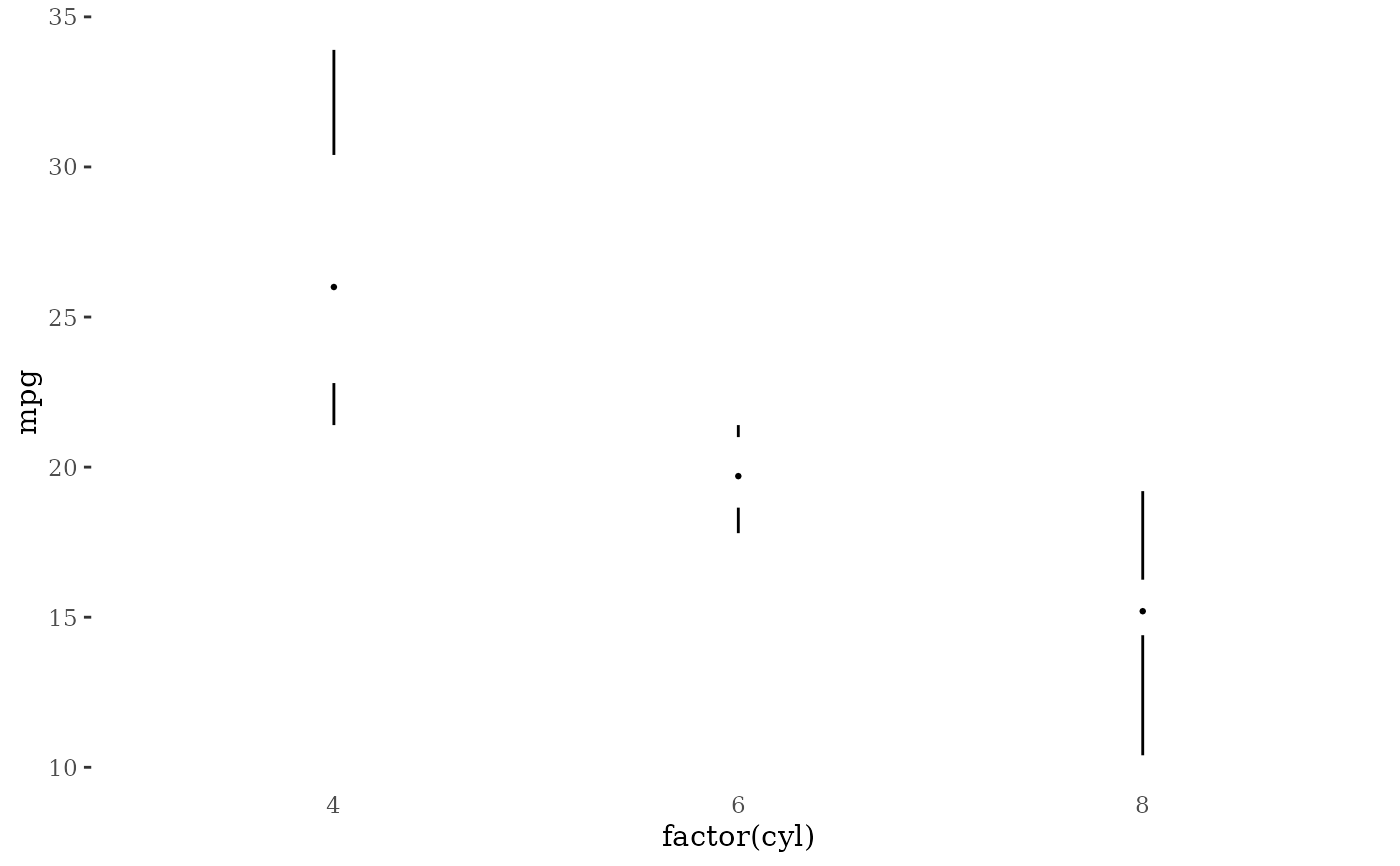Edward Tufte's revisions of the box plot as described in The Visual Display of Quantitative Information. This functions provides several box plot variants:

• A point indicating the median, a gap indicating the interquartile range, and lines for whiskers.

• An offset line indicating the interquartile range and a gap indicating the median.

• A line indicating the interquartile range, a gap indicating the median, and points indicating the minimum and maximum values

• A wide line indicating the interquartile range, a gap indicating the median, and lines indicating the minimum and maximum.

geom_tufteboxplot(
mapping = NULL,
data = NULL,
stat = "fivenumber",
position = "dodge",
outlier.colour = "black",
outlier.shape = 19,
outlier.size = 1.5,
outlier.stroke = 0.5,
voffset = 0.01,
hoffset = 0.005,
na.rm = FALSE,
show.legend = NA,
inherit.aes = TRUE,
median.type = "point",
whisker.type = "line",
...
)

## Arguments

mapping Set of aesthetic mappings created by aes() or aes_(). If specified and inherit.aes = TRUE (the default), it is combined with the default mapping at the top level of the plot. You must supply mapping if there is no plot mapping. The data to be displayed in this layer. There are three options: If NULL, the default, the data is inherited from the plot data as specified in the call to ggplot(). A data.frame, or other object, will override the plot data. All objects will be fortified to produce a data frame. See fortify() for which variables will be created. A function will be called with a single argument, the plot data. The return value must be a data.frame, and will be used as the layer data. A function can be created from a formula (e.g. ~ head(.x, 10)). The statistical transformation to use on the data for this layer, as a string. Position adjustment, either as a string, or the result of a call to a position adjustment function. colour for outlying points shape of outlying points size of outlying points stroke for outlying points controls the size of the gap in the line representing the median when median.type = 'line'. This is a fraction of the range of y. controls how much the interquartile line is offset from the whiskers when median.type = 'line'. This is a fraction of the range of x. If FALSE, the default, missing values are removed with a warning. If TRUE, missing values are silently removed. logical. Should this layer be included in the legends? NA, the default, includes if any aesthetics are mapped. FALSE never includes, and TRUE always includes. It can also be a named logical vector to finely select the aesthetics to display. If FALSE, overrides the default aesthetics, rather than combining with them. This is most useful for helper functions that define both data and aesthetics and shouldn't inherit behaviour from the default plot specification, e.g. borders(). If 'point', then the median is represented by a point, and the interquartile range by a gap in the line. If median.type='line', then the interquartile range is represented by a line, possibly offset, and the median by a gap in the line. If 'line', then whiskers are represented by lines. If 'point', then whiskers are represented by points at ymin and ymax. Other arguments passed on to layer(). These are often aesthetics, used to set an aesthetic to a fixed value, like colour = "red" or size = 3. They may also be parameters to the paired geom/stat.

## Aesthetics

• x [required]

• y [required]

• colour

• size

• linetype

• shape

• fill

• alpha

Tufte, Edward R. (2001) The Visual Display of Quantitative Information, Chapter 6.

McGill, R., Tukey, J. W. and Larsen, W. A. (1978) Variations of box plots. The American Statistician 32, 12-16.

geom_boxplot()

Other geom tufte: geom_rangeframe()

## Examples

library("ggplot2")

p <- ggplot(mtcars, aes(factor(cyl), mpg))
# with a point for the median and lines for whiskers
p + geom_tufteboxplot()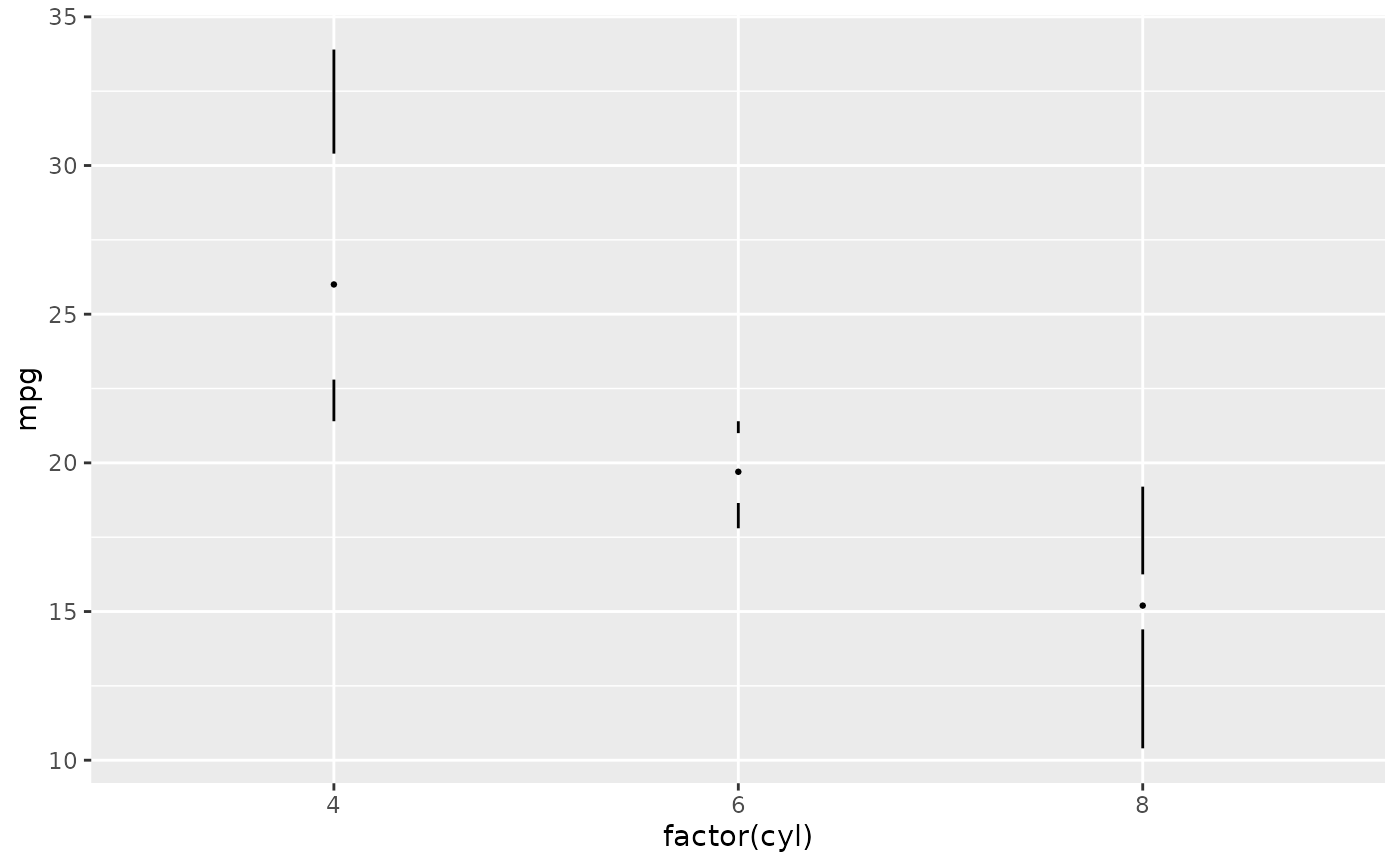# with a line for the interquartile range and points for whiskers
p + geom_tufteboxplot(median.type = "line", whisker.type = "point", hoffset = 0)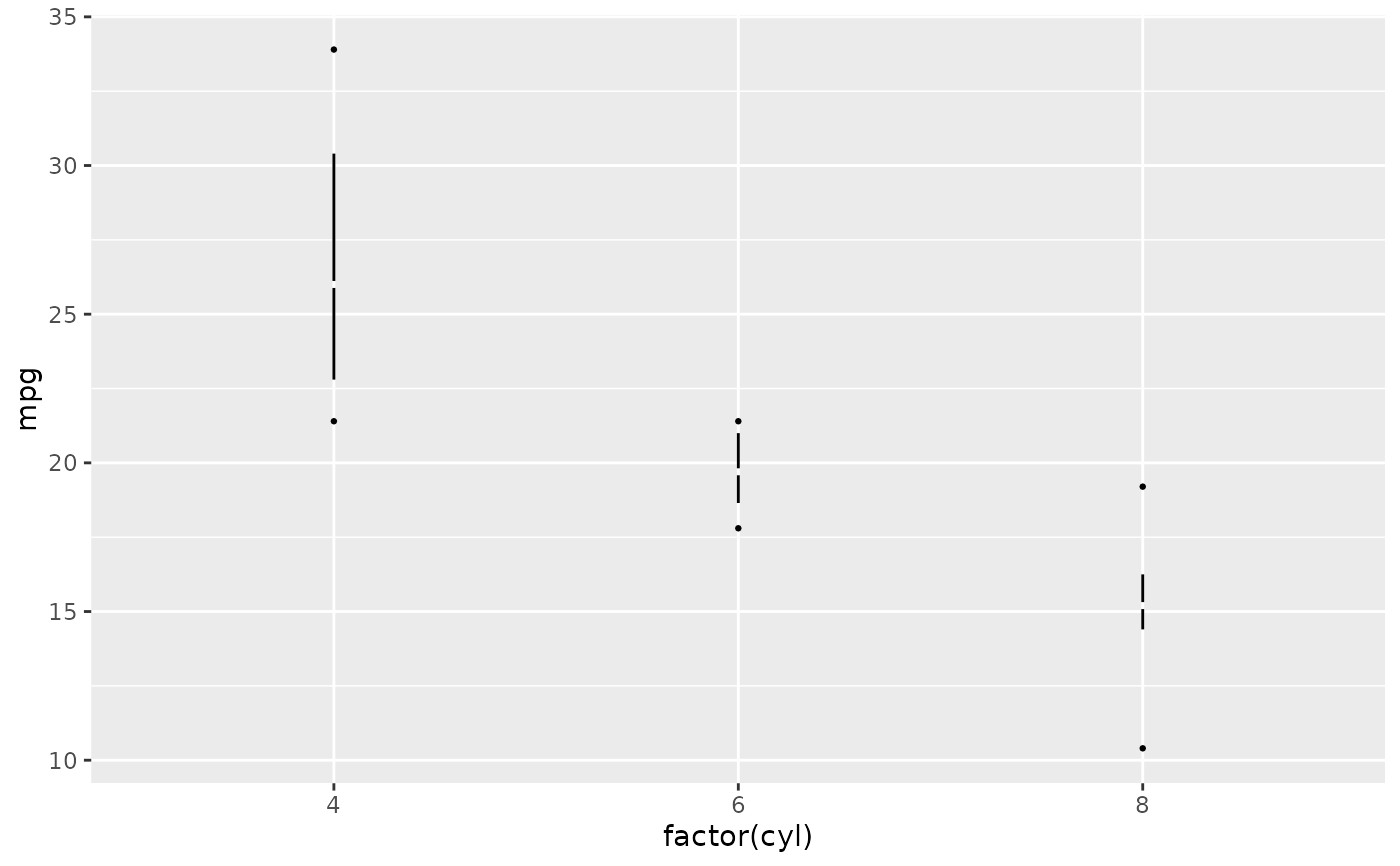# with a wide line for the interquartile range and lines for whiskers
p + geom_tufteboxplot(median.type = "line", hoffset = 0, width = 3)
#> Warning: position_dodge requires non-overlapping x intervals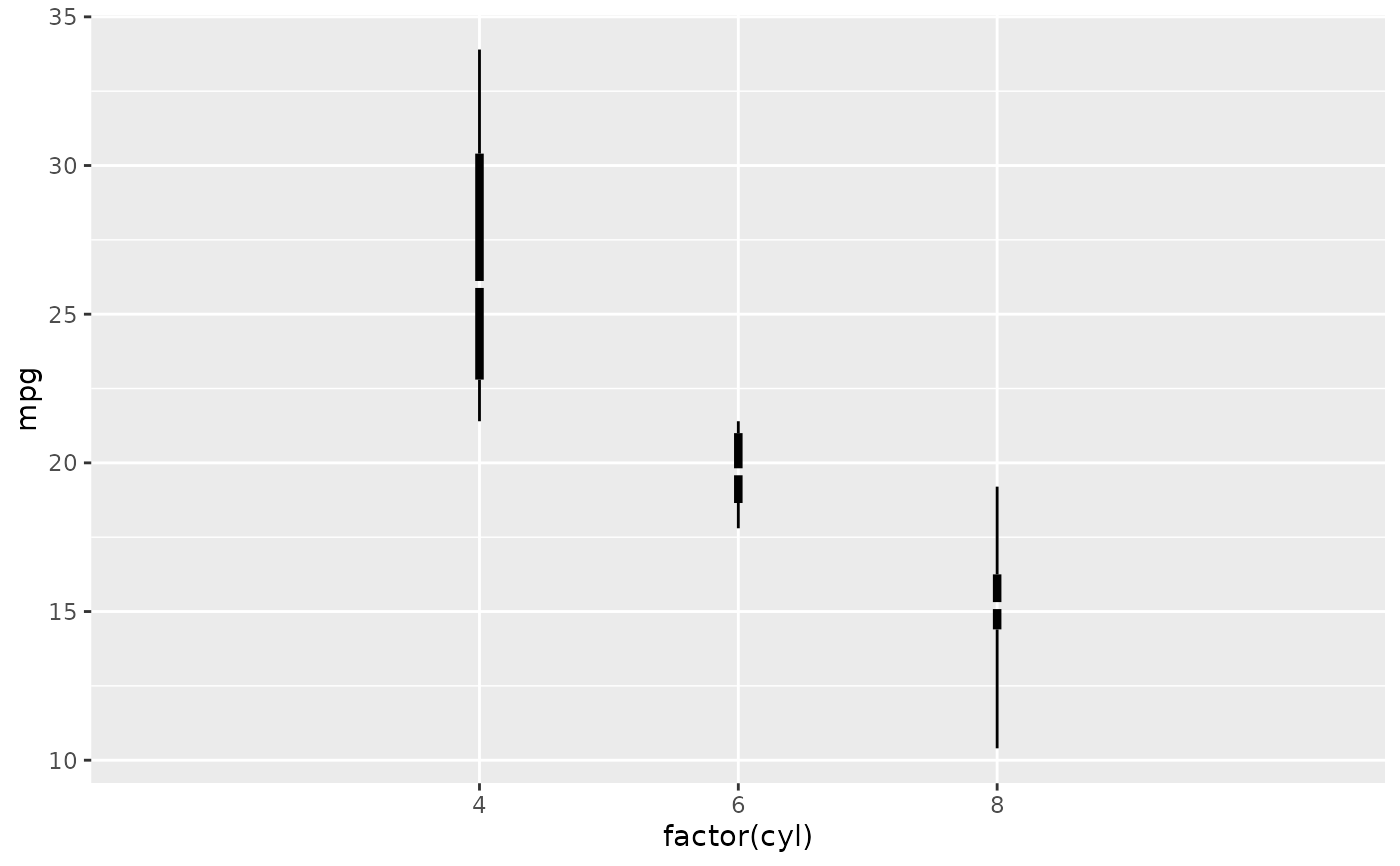# with an offset line for the interquartile range and lines for whiskers
p + geom_tufteboxplot(median.type = "line")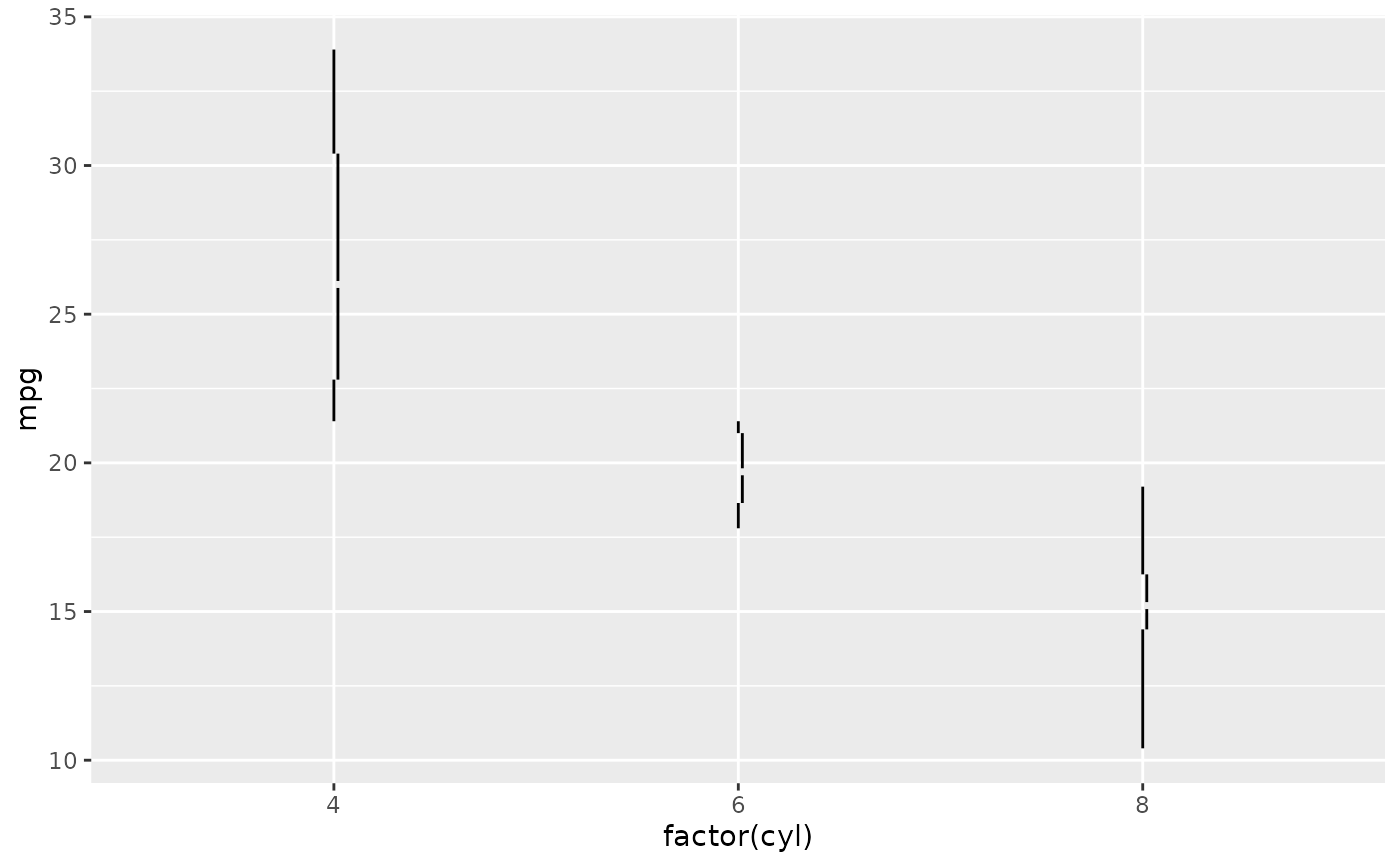# combined with theme_tufte
p + geom_tufteboxplot() +
theme_tufte() +
theme(axis.ticks.x = element_blank())